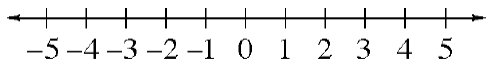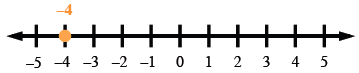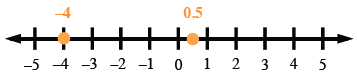### Home > MC2 > Chapter 1 > Lesson 1.1.2 > Problem1-14

1-14.

Copy the number line below onto your paper. Place a point on the number line and label the point for each of the following numbers: $−4$, $3$, $0.5$, $−3.5$ , $0$, $1$, $−2$ , $5$. .Here is one example.

Here is another example. Can you place and label the rest of the points?Explore using the eTool below.
Click on the link at the right for the full eTool version: MC2 1-14 HW eTool